Friday February 3, 2023
Latest:
349 total views

It’s so vital that fifth grade math students master key concepts like fractions and decimals as they prepare to advance to high math classes. These games can help with those skills, along with coordinate planes, calculating volume, and more. Choose a few to try in your classroom!

(Just a heads up, WeAreTeachers may collect a share of sales from the links on this page. We only recommend items our team loves!)

## 1. Flip cards to compare decimals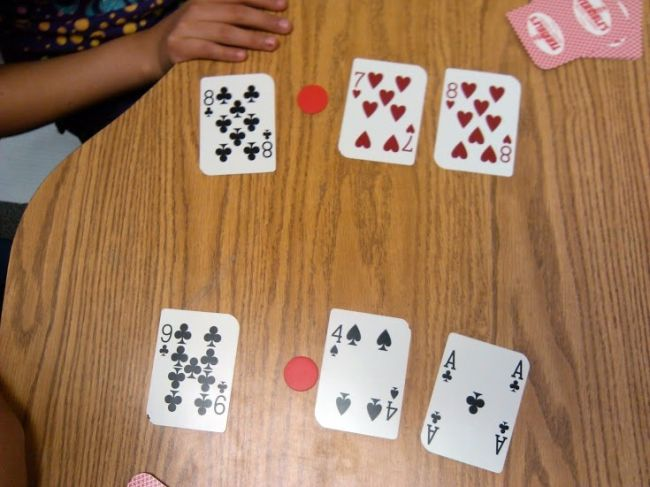In this version of War, students flip three cards and place a chip as a decimal point. Then, they compare their cards to see whose number is greater. The winner takes all the cards.

## 2. Make a Buck to get practice adding decimals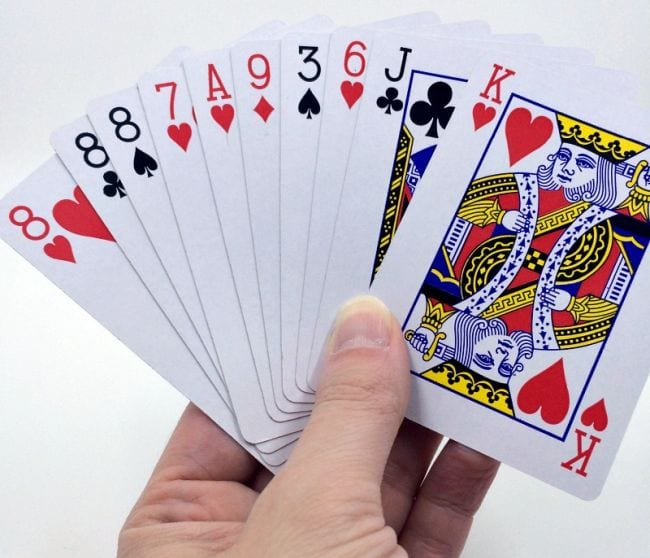Money is a useful way to give decimals real-life application. In this game, students use playing cards to represent amounts and work to get their hands to equal exactly one dollar. (Find more math card games here.)

## 3. Have a decimal snowball fight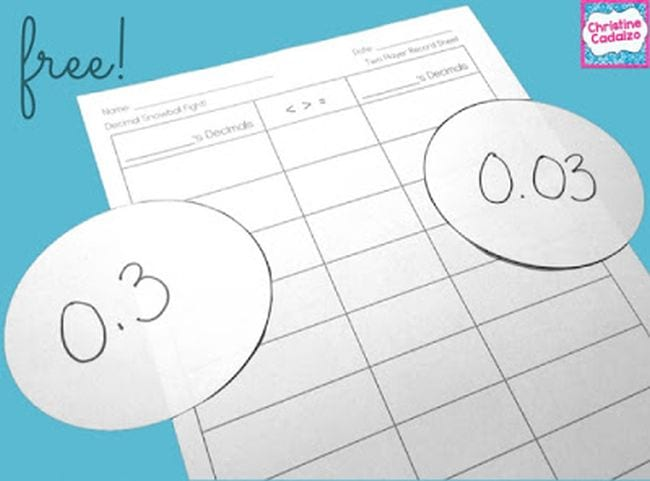Each student receives a stack of decimal “snowballs.” They each flip one over and compare their numbers. The student with the larger number keeps both snowballs.

## 4. Turn students into decimals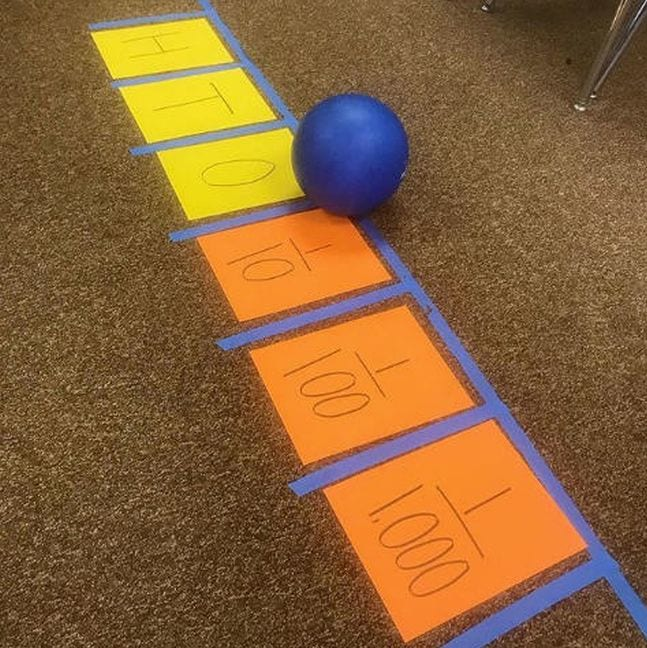Even fifth grade math students aren’t too old to enjoy human number lines! Lay out the line on your floor, using a playground ball for a decimal point. Then try this: choose ten students and give them each a large card numbered from 0-9. Have another student secretly write down a number to the thousandths place, then provide instructions to get the numbers to stand in the right places. For instance, “Number 3 is in the hundreds place. Number 6 is in the hundredths place.” See how quickly students can get into the right place in line!

Source: @desire_to_inspire

## 5. Try to guess Where Is It?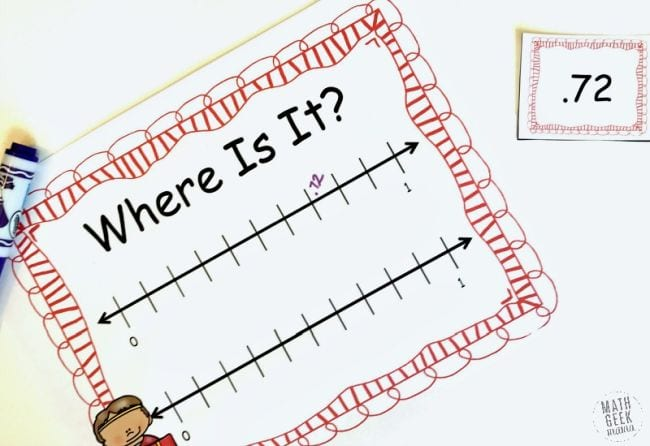One player secretly plots a decimal on the line. The other player guesses a decimal and plots it on their own number line. The first player tells them whether their guess is larger or smaller than the correct number. The players keep narrowing it down until they’ve correctly identified each other’s numbers.

## 6. Add and subtract decimals to win the game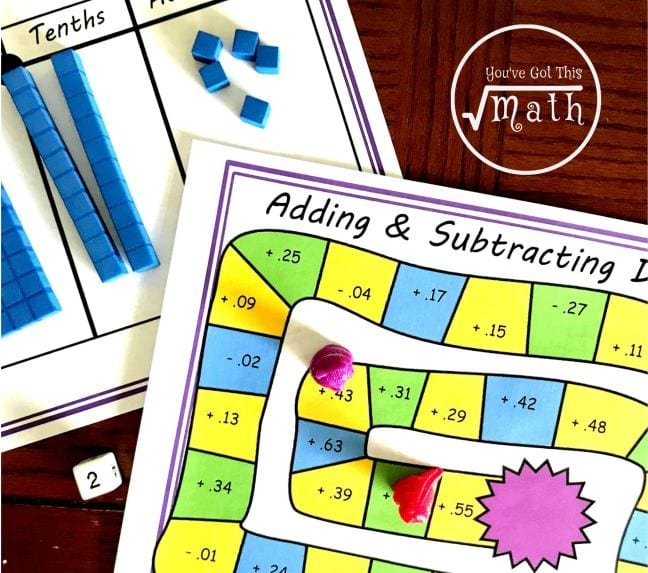Print this free game at the link, then have fifth grade math students roll the dice, move their pieces, and add or subtract the numbers shown. The other student checks their answer on a calculator. If they’re correct, they stay there. If not, they move back. For extra practice, they can use base 10 blocks too.

## 7. Give Bingo a decimals twist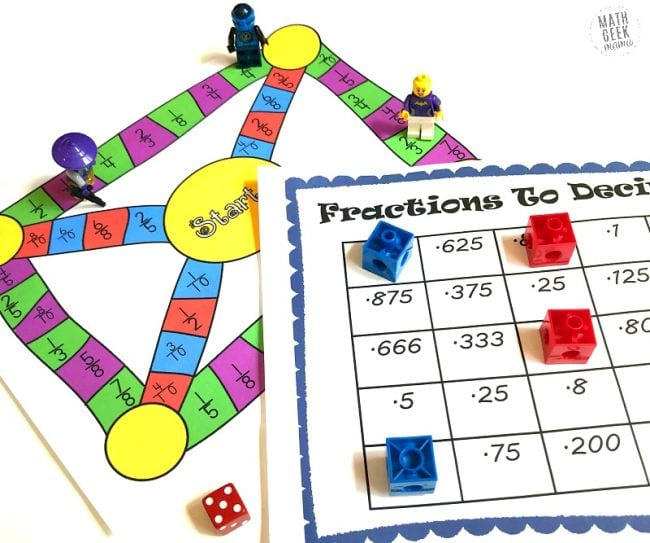Convert fractions to decimals and see who can be the first to Bingo in this free printable game.

## 8. Grab some spoons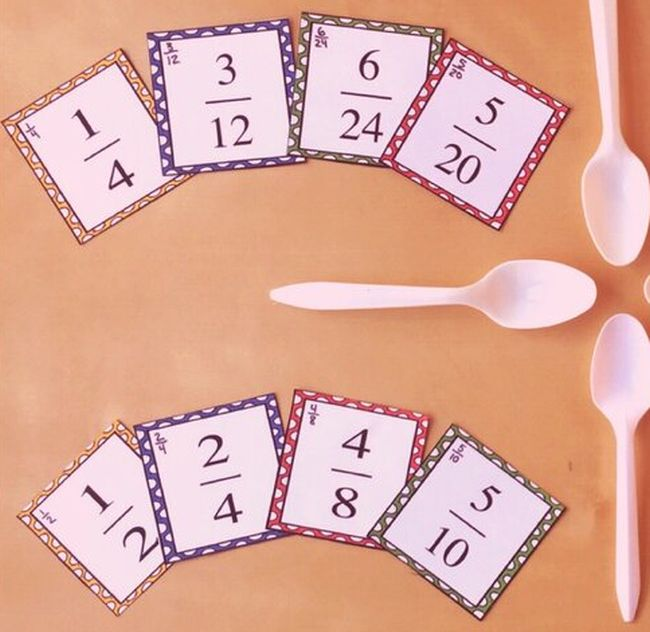If you’ve played Spoons before, you know the drill. Match four of a kind (this time using free printable fraction cards found at the link below), and you get to grab for a spoon—and so does everyone else! The loser takes one letter of the word SPOON, and play continues.

## 9. Use counters for fraction practice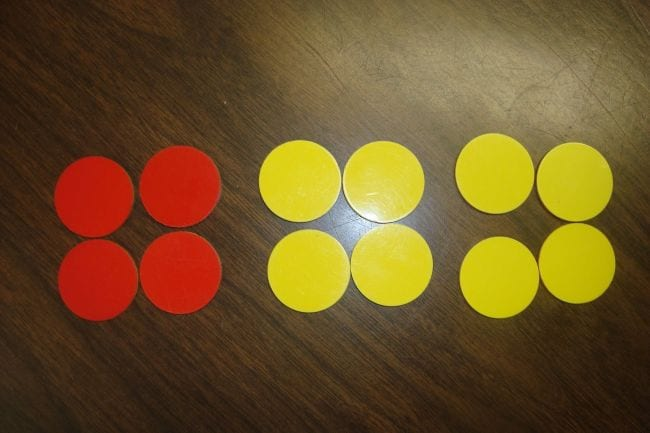Grab a handful of counter chips of two different colors and lay them out. Then challenge students to a variety of fraction activities: what fraction do they make? What are the equivalent fractions? Repeat the activity a few times and add, subtract, multiply, or divide the fractions.

## 10. Multiply and compare fractions with dominoes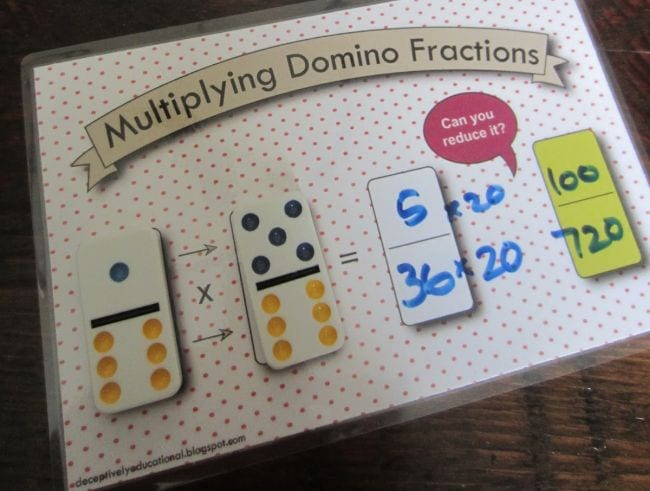Fifth grade math students do a lot of work with fractions, and dominoes are perfect manipulatives! In this simple game, each student draws two dominoes. They multiply and reduce, then see who has the larger number.

## 11. Race on a number line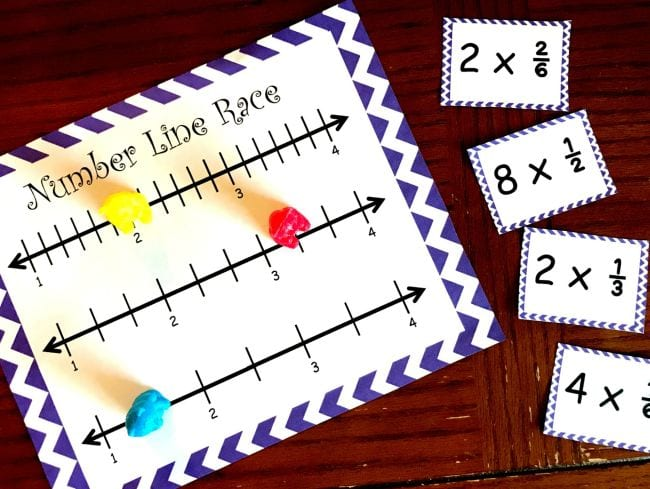This free printable game gives kids practice multiplying fractions by a whole number. Grab it at the link. (Here are more number line activities for all ages.)

## 12. Play Fraction War with dominoes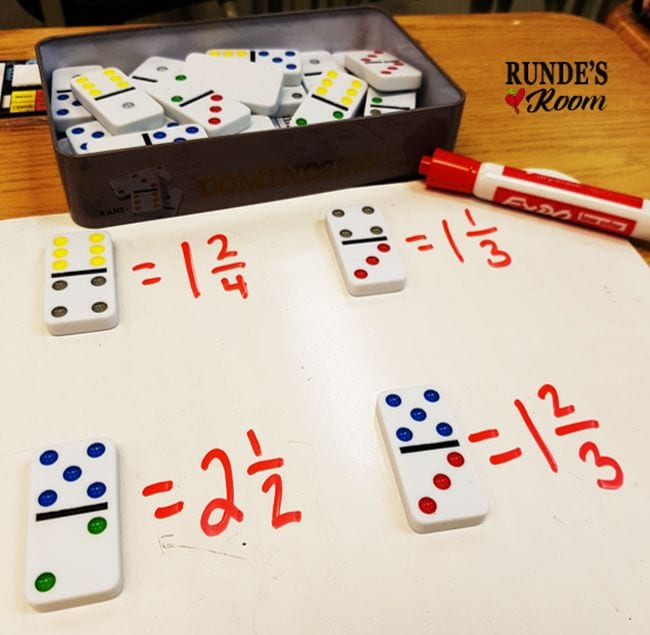Each player flips a domino and turns it so the larger number is on top, making an improper fraction. Then they write the mixed number and reduce it if needed. The player with the larger number keeps both dominoes.

Source: Runde’s Room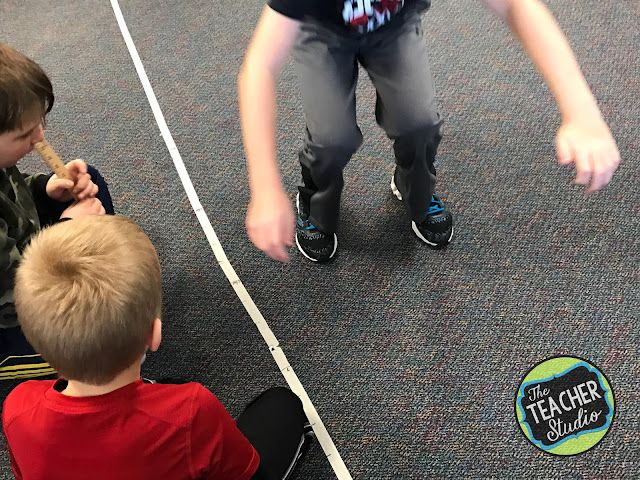This is such a fun way to practice gathering data and using it to make line plots! A long jump contest gets kids up and moving. You can also measure your wrists or your feet.

## 14. Build with pattern blocks to be the First to Six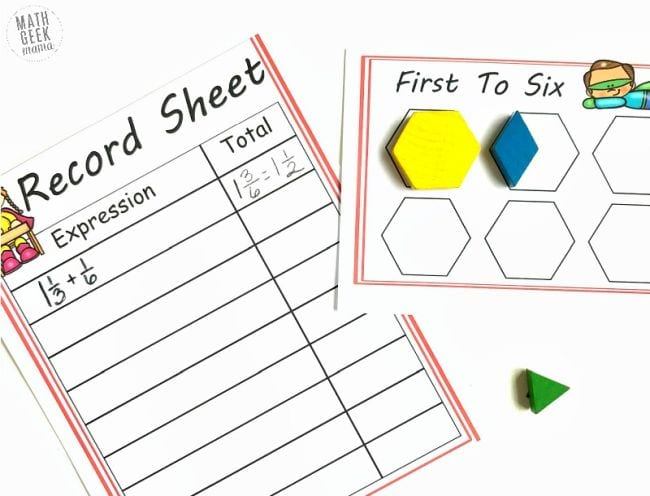Pull out your pattern blocks and have fifth grade math students use them as they work on adding mixed numbers.

## 15. Write equations to play Can You Make It?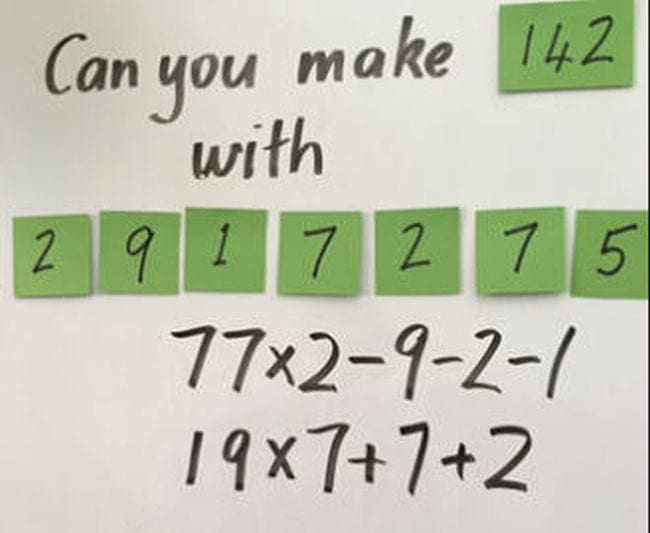All you need for this simple fifth grade math game are some sticky notes. Set a target number and provide numerals to work with. Challenge kids to use a variety of operations, including brackets.

## 16. Build with LEGO bricks to measure volume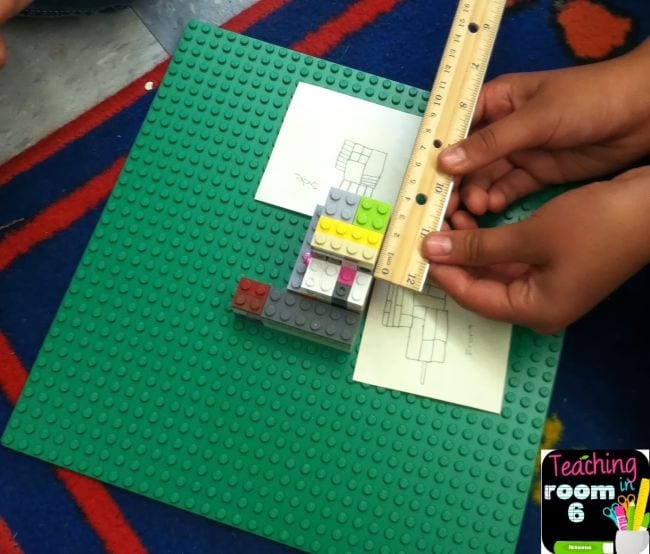Fifth grade math students encounter volume for the first time. LEGO bricks can be a great way to introduce the subject and help them see how it works.

## 17. Play Two Truths and a Lie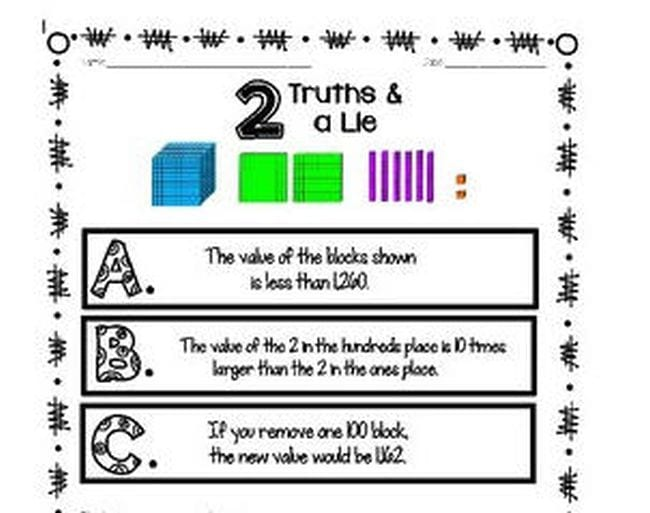Working on volume? Pose a “Two Truths and a Lie” puzzle, then challenge students to come up with their own!

## 18. Write the room for volume practice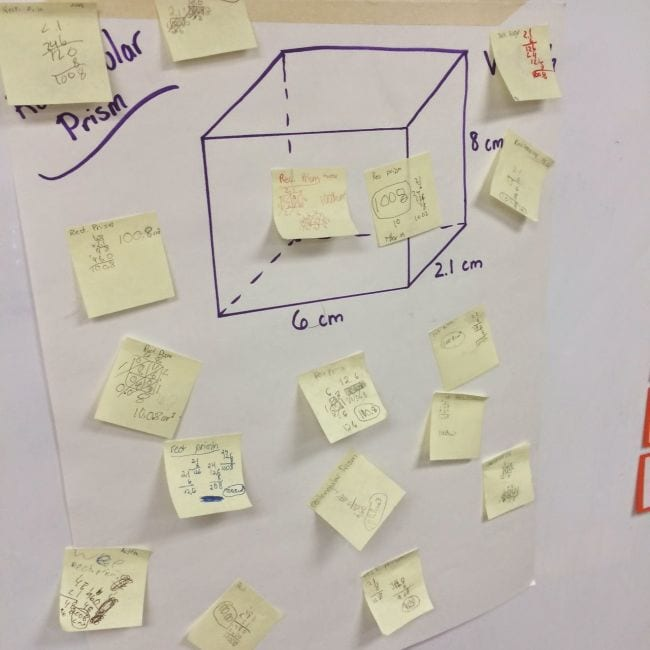Post a series of figures around the room for students to find. Ask them to calculate the volume (alone or in groups) and post their answer on sticky notes that shows their work. (Check out these other ways to Write the Room.)

## 19. Connect Four in a coordinate plane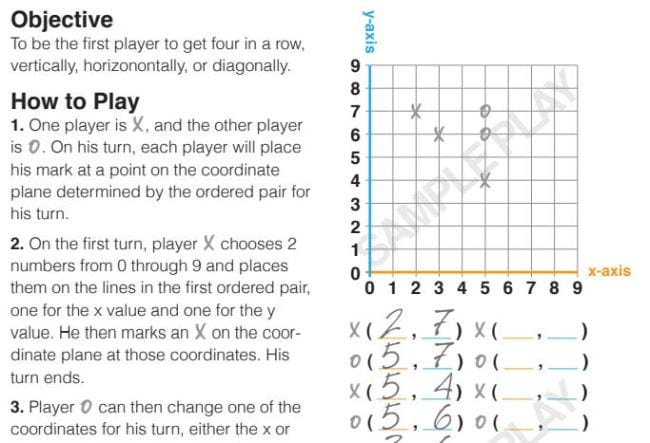Coordinate planes are another new concept in fifth grade math. This simple game helps kids learn how they work as they try to be the first to graph four in a row.

## 20. Track minifigs on a coordinate plane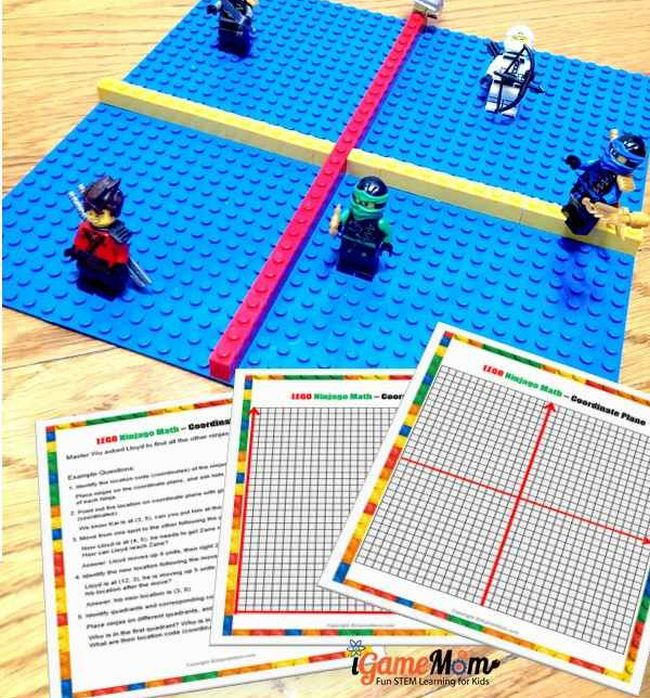Here’s another way to use LEGO bricks for fifth grade math. Kids locate the hidden minifigs using coded coordinates. So cool!

## 21. Reveal the truth about Battleship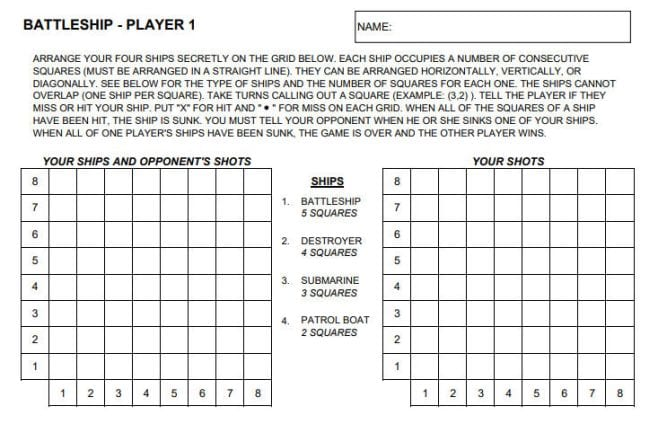Remember when you realized that the game Battleship was really all about coordinate planes? Time to share that with your fifth grade math students! Play the real game, or get free printables to use at the link below.

## 22. Make a human coordinate plane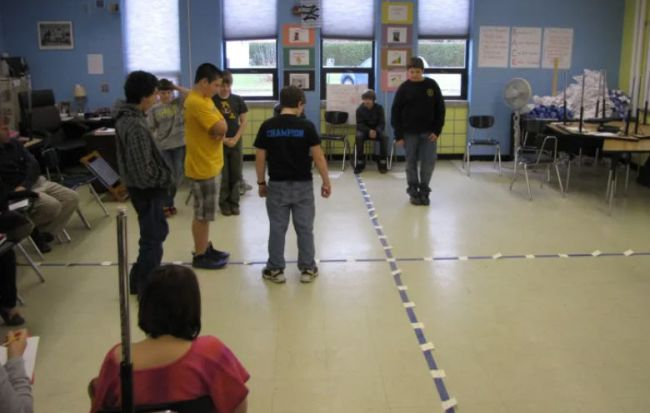Does your classroom have square floor tiles? Use them to make a life-size coordinate plane! Then have kids stand in one spot and jump to a new one. When they arrive, they must announce their new coordinates. You can also call out instructions like “Move ahead two on the X-axis and back three on the Y-axis.” Kids will think and move at the same time, reinforcing the learning.

## 23. Solve a mystery picture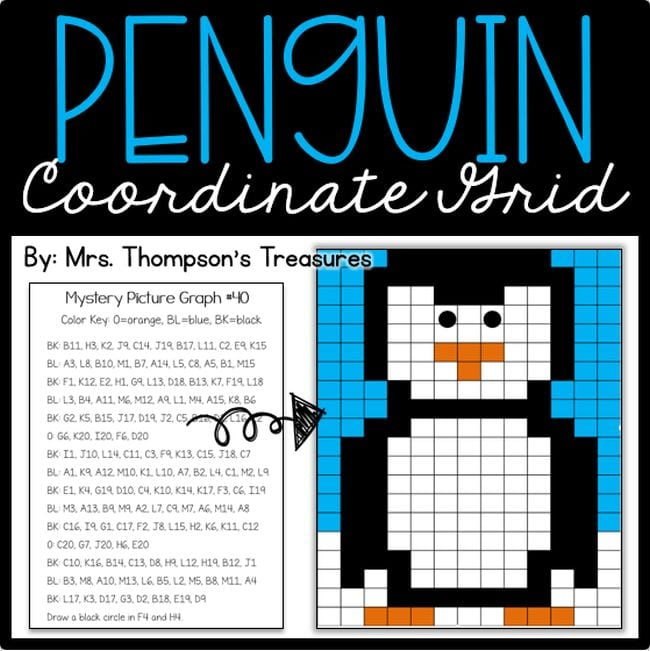Coordinate plane mystery pictures are fun and very popular. You can find lots of options available on the web. Try this for free at the link.

### News

March is Women’s History Month, coinciding with International Women’s Day on March 8. It’s a time to recognize the…